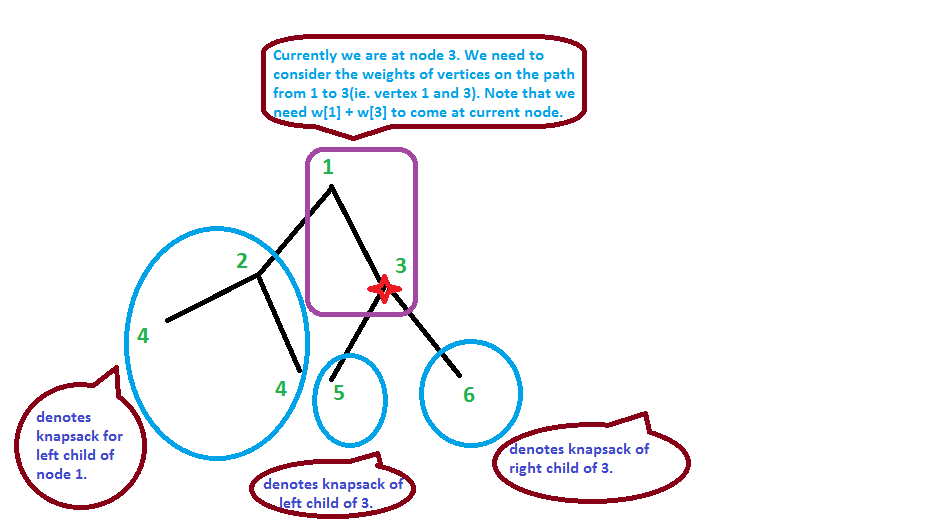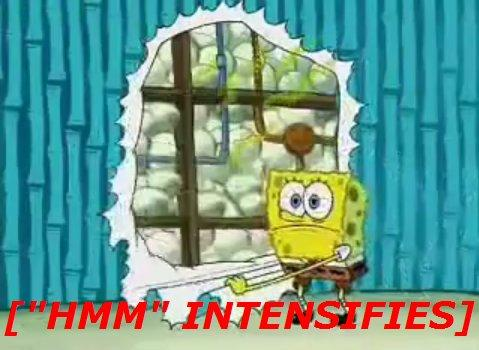You are not logged in. Please login at www.codechef.com to post your questions!

×

# RRTREE2 - editorial

Author: Roman Rubanenko
Editorialist: Praveen Dhinwa

Medium-Hard

# PREREQUISITES:

knapsack, graph theory, tree, dfs.

# PROBLEM:

You are given a rooted tree with N vertices. Vertices are numbered from 1 to N and vertex 1 is the root of the tree. A positive integer Wi is assigned to every node. Consider following code:

 Integer sum := 0; Array of boolean marked := {false, false, false, ..., false}; Procedure Dfs(Integer v) Begin sum := sum + Wv; marked[sum] := true; For each node u that v is a parent of u do Begin Dfs(u); End; End; 

One can notice that the state of marked[] array depends on the order vertices u processed. You are to check for every integer number s from 1 to the sum of $W_i$ whether there exists some order of viewing children in Dfs that marked[s] = true.

# Explanation

How is marked array related to dfs?

Note that when we do dfs, we chose the next node to go from the current node. While choosing the next node, we have freedom of choosing any child of current node as the next vertex to do dfs from. This freedom will effect the global variable sum. Hence state of marked[] array will depend on the order of vertices processed in the dfs.

Is there some nice property of dfs which can come handy?

Note that during dfs, if you are currently at vertex v, then following cases can occur.

• You have first time come at vertex v by using a direct path from root to current vertex v.
• You have visited some of the children subtrees rooted at vertex v. Note that if you are currently at vertex v after visiting them, then it wont happen that some subtree is partially visited (it will be either fully visited or not visited at all). It is due to property of dfs, that it will keep going down until it finds a possible way of going.

Great, Can you we use it somehow in our problem?

Let's store boolean array for global answer. The array size is equal to sum of all the weights, it will contain 1 if corresponding weight is achievable, otherwise it will contain zero.

So now let's do a dfs from the root and store current knapsack that shows what weights we can get at the moment of visiting current vertex. When we are at some vertex, let's add items with weights equal to the sizes of all the children of this node. By subtree size, I mean the sum of weight of all the vertices who lie in the subtree.

When we go down to one of these children, we should delete it's subtree size, go down, return and add the value back.

Yes, I understood it, Can you provide some pseudo code?

Pseudo Code
 void dfs(from, curSum): // update curSum by weight of our current vertex i.e. from. curSum += w[from] updateGlobalAnswer(curSum); // now add all the subtree sizes of its chilren to the knapsack. for v in child(from): addItem(subtreeSize[v]); // for each children, go to them, before going to them, delete the // subtree size of that children from the knapsack. // After the visit of that vertex, re-add the subtree size. for v in child(from): deleteItem(subtreeSize[v]); dfs(v, curSum); addItem(subtreeSize); // now finally delete all the subtree sizes that you had added initially. for v in child(from): deleteItem(subtreeSize[v]); 

Can you take one example?

Yes, I will try to explain you concept of the knapsack's. Let us have a look at this picture.When we are node 3, we have value of curSum equal to weight of vertices on the path from root to current vertex (ie. vertex 1 and 3). Note that There are following ways of coming at node 3. - We have directly come to node 3(ie. from 1 to 3) - We have visited the left subtree of 1 and then come directly to node 3. - We might have visited the left subtree of 1 and we have come from 1 to 3, then from 3, we have visited one of the subtrees of 3, and have come back to 3.

Note that currently, the value of variable sum will be atleast weight of path from root to current (vertex 1 and 3). Other than that, it can be any combination of left subtree of 1, left and right subtree of 3. So we can consider these three subtree's as knapsack's.

If you have not understand the details upto now. Please try to create idea for non-recursive solution (without using dfs). You will get an exact idea about which of the subtrees has to deleted and which of them have to be considered in the knapsack.

Yes, I got that. But how will I add and delete items from the knapsack?

Yes, So we should be able to handle adding/deleting an item from the knapsack.

Let's store number of ways we can get a particular weight rather than storing simple boolean value (whether the weight is achievable or not?). It will allow us to add/delete items. So we can obtain a particular weight if corresponding number of ways is greater than zero. Since the number of ways of obtaining a particular weight can be very huge, we have to store it using modulos some number. Now it is better to use more than one modulo's, It will provide us more accuracy. This is like hashing in some way. tourist's solution uses modulo 10^9 + 7 and 10^9 + 9. Tester's solution contains more than 2 modulos.

Can you please explain idea of adding a weight in knapsack with some codes?

Yes, if we are adding a weight w into the knapsack. The number of ways of getting value V increase by number of ways of getting weight V - w.

Pseudo Code
 // here sum denotes maximum possible value achievable by the knapsack. // note that i will go upto w. Because other then i - w will be negative. // You can note that order of visting is not from w to sum, but from sum to w. // because we can write recurrence in new form like this. // let new_dp be the new knapsack after adding the element. // new_dp[i] = dp[i] + dp[i - w]. // So you can simply notice that if you go from right to left, you can update the // current dp[i] by new_dp[i], because we are not going to use // that index. But this is not true when we go from left to right. void addItem(int w){ for(int i = sum; i >= w; i--){ dp[i] += dp[i-w]; if(dp[i]>MOD) dp[i]-=MOD; } } } 

Great, Can you please idea of removing a weight in knapsack with some codes?

Yes, if we are removing a weight w from the knapsack. The number of ways of getting value V will decrese by number of ways of getting weight V - w because these were precisely the cases where we had weight V including the current weight w.

Pseudo Code
 void deleteItem(int w){ for(int i = w; i <= sum; i++){ dp[i] -= dp[i-w]; if(dp[i]<0) dp[i]+=MOD; } } 

Cool, I understood everything. Can we go on the complexity part?

Complexity:
Complexity of solution is O(n * W). Because during the dfs, we have to update the knapsack each time. Updating the knapsack takes O(W) times. So total time required is O(n * W). Here W is sum of weights of all the n nodes.

# AUTHOR'S and TESTER'S SOLUTIONS:

This question is marked "community wiki".

asked 21 Jul '14, 01:042.5k53137170
accept rate: 20%

the link to tourist's solution is wrong

(21 Jul '14, 18:46) 3★

 5 You know what's the complexity of my solution (fastest AC'd in the contest)? O(WN^2) I can't believe the weak tests weren't intentional. Because there's no way anyone could (unintentionally) use a maxtest with a star in a graph problem. This test: 500 200 200 ... 200 (500x) 1 1 ... 1 (500x, because 499 is an ugly number)  takes my code (again, the same fastest AC) 1.5 minute locally. It could TLE me into oblivion, and I was aware of it when I was submitting, about a minute before the contest ended. My thought process was something like "oh well, nothing to lose by submitting this slow shit". And then, mfw I saw it at the top of the Best submissions listanswered 21 Jul '14, 01:167★xellos0 5.9k●5●43●93 accept rate: 10% your memes are creative :) (21 Jul '14, 01:25) It was a big mistake of course. We were too much busy with the correctness of the solution that we have forgot to add some strong cases :( :'( (21 Jul '14, 04:46) shiplu2★
 5 [Edit : If you looked at xellos0 answer, you can skip this answer] This O(n * n * W) solution is supposed to fail. http://www.codechef.com/viewsolution/4360304 Are the test cases weak? I am only considering addition into knapsack, doing it O(childCount) times. So it is O(childCount * childCount) which has to time out. I used a different approach in contest which is kind of decomposition when number of one bits in current array are low and that had bad runtime than this n * n * W solution. answered 21 Jul '14, 01:252.2k●7●26●33 accept rate: 20%
 1 I don't understand the part of deleting and adding items in knapsack. How it is done? ' But how will I add and delete items from the knapsack? ' can someone please elaborate ? answered 21 Jul '14, 18:52366●2●7●11 accept rate: 0%
 toggle preview community wiki:
Preview

### Follow this question

By Email:

Once you sign in you will be able to subscribe for any updates here

Markdown Basics

• *italic* or _italic_
• **bold** or __bold__
• image?![alt text](/path/img.jpg "title")
• numbered list: 1. Foo 2. Bar
• to add a line break simply add two spaces to where you would like the new line to be.
• basic HTML tags are also supported
• mathemetical formulas in Latex between \$ symbol

Question tags:

×15,852
×1,299
×733
×86
×15

question asked: 21 Jul '14, 01:04

question was seen: 9,403 times

last updated: 21 Jul '14, 21:31